# Samacheer Kalvi Books: Tamilnadu State Board Text Books Solutions

## Samacheer Kalvi 8th Maths Chapter 1 Numbers Notes PDF Download: Tamil Nadu STD 8th Maths Chapter 1 Numbers NotesSamacheer Kalvi 8th Maths Chapter 1 Numbers Notes PDF Download: Tamil Nadu STD 8th Maths Chapter 1 Numbers Notes

We bring to you specially curated Samacheer Kalvi 8th Maths Chapter 1 Numbers Notes PDF which have been prepared by our subject experts after carefully following the trend of the exam in the last few years. The notes will not only serve for revision purposes, but also will have several cuts and easy methods to go about a difficult problem.

 Board Tamilnadu Board Study Material Notes Class Samacheer Kalvi 8th Maths Subject 8th Maths Chapter Chapter 1 Numbers Format PDF Provider Samacheer Kalvi Books

## How to Download Samacheer Kalvi 8th Maths Chapter 1 Numbers Notes PDFs?

2. Click on the Samacheer Kalvi 8th Maths Notes PDF.
3. Look for your preferred subject.
4. Now download the Samacheer Kalvi 8th Maths Chapter 1 Numbers notes PDF.

## Tamilnadu Samacheer Kalvi 8th Maths Solutions Chapter 1 Numbers InText Questions

Recap Exercise (Text Book Page No. 3)

Question 1.
The simplest form of 125200 is
125200=125÷25200÷25=58
= 58

Question 2.
Which of the following is not an equivalent fraction of 812 ?
(A) 23
(B) 1624
(C) 3260
(D) 2436
(C) 3260
812=8+412÷4=23
812=8×212×2=1624
812=8×312×3=2436
But 3260=32÷560÷5=6.412
∴ 3260 is not equivalent fraction of 812

Question 3.
Which is bigger 89 or 45 ?
LCM of 5 and 9 = 4589 is bigger than 45

Question 4.
LCM of 5, 8, 10 = 5 × 2 × 4
= 40Question 5.
Simplify: 18−(16−14)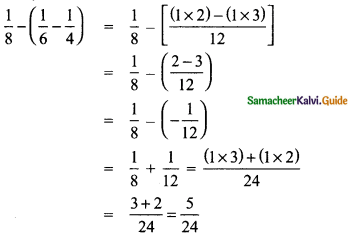Question 6.
Multiply: 235 and 147.
235×147=135×117=14335=4335

Question 7.
Divide 736 by 3581.
736+3581=736×8135=920

Question 8.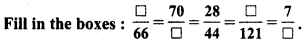Question 9.
In a city 720 of the population are women and 14 are children. Find the fraction of the population of men.
Let the total population = 1
Population of men = Total population – Women – Children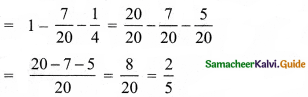∴ Population of men = 25

Question 10.
Represent (12+14) by a diagram.Try These (Text Book Page No. 3)

Question 1.
Is the number -7 a rational number? Why?
A rational number, Because – 7 = −142=pq

Question 2.
Write any 6 rational numbers between 0 and 1.
12,13,14,15,16,17

Try These (Text Book Page No. 4)

Write the decimal forms of the following rational numbers:

Question 1.
45
45=4×205×20=80100=0.80

Question 2.
625
625=6×425×4=24100=0.24

Question 3.
4861000
4861000 = 0.486

Question 4.
19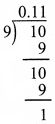19 = 0.11….

Question 5.
314314 = 134 = 3.25

Question 6.
−235−235 = −135 = -2.6

Try These (Text Book Page No. 6)

Question 1.
73=?9=49?=−21?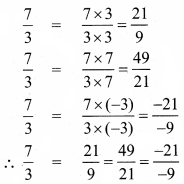Question 2.
−25=?10=6?=−8?Try These (Text Book Page No. 7)

Question 1.
Which of the following pairs represents equivalent rational numbers?
(i) −64,18−12
(ii) −4−20,1−5
(iii) −12−17,6085
(i) −64,18−12
−64=−6×34×3=−1812
∴ −64 equivalent to −1812

(ii) −4−20,1−5
−4−20=−4÷(−4)−20÷(−4)=15≠−15
∴ −4−20 equivalent to 1−5

(iii) −12−17,6085
−12−17=−12x−5−17x−5=6085
∴ −12−17 equivalent to 6085

Question 2.
Find the standard form of
(i) 36−96
(ii) −56−72
(iii) 2718
(i) 36−96
= −36÷1296÷12=−38

(ii) −56−72
= −56÷(−8)−72÷(−8)=79

(iii) 2718
= 1918=112

Question 3.
Mark the following rational numbers on a number line.
(i) −23
−23 lies betveen 0 and -1.
T?ìe unit part between O and —lis divided into 3 equal parts and second part is taken.(ii) −8−5
−8−5 = 135
135 lies between I and 2, The unit part between I and 2 is divided into 5 equal parts and the third part is taken.(iii) 5−4
5−4 = −54 = −114
−114 lies between -1 and -2. The unit part between -1 and -2 is divided into four equal parts and the first part is taken.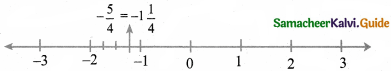Think (Text Book Page No. 15)

Is zero a rational number? If so, what is its additive inverse
Yes zero a rational number Additive inverse of zero is zero.

Think (Text Book Page No. 16)

What is the multiplicative inverse of 1 and -1?
Multiplicative inverse of 1 is 1 and -1 is -1.

Try These (Text Book Page No. 16)

Divide
(i) −73 by 5
(ii) 5 by −73
(iii) −73 by 356
(i) −73 by 5(ii) 5 by −73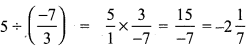(iii) −73 by 356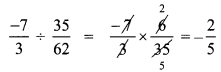Try These (Text Book Page No. 20)

The closure property on integers holds for subtraction and not for division. What about rational numbers? Verify.
Let 0 and 12 te two rational numbers 0 – 12 = –12 is a rational numter
∴ Closure property for subtraction holds for rational numbers.
But consider the two rational number 52 and 0.
52 + 0 = 52×0=50
Here denominator = 0 and it is not a rational number.
∴ Closure property is not true for division of rational numbers.

Try These (Text Book Page No. 22)

(i) Is 35−78=78−35 ?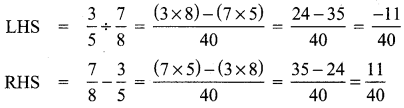LHS ≠ RHS
∴ 35÷78 ≠ 78−35
∴ Subtraction of rational numbers is not commutative.

(ii) 35÷78=78÷53? So, what do you conclude?LHS ≠ RHS
∴ 35÷78 ≠ 78÷53
∴ Commutative property not hold good br division of rational numbers.

Try This (Text Book Page No. 22)

Check whether associative property holds for subtraction and division.
Consider for rational numbers 23,12 and 34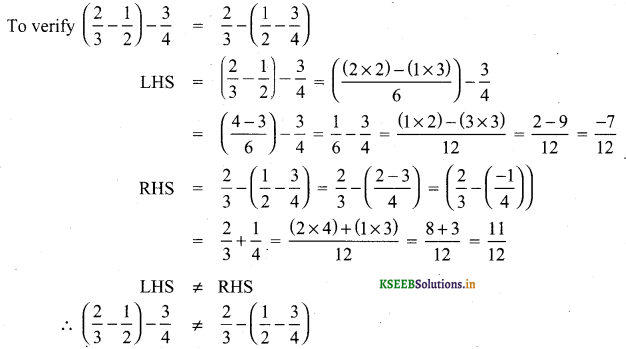∴ Associative property not holds for subraction of rational numbers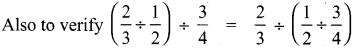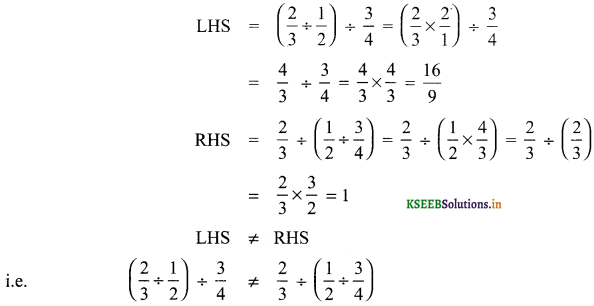∴ Associative property not holds for division of rational numbers

Try This (Text Book Page No. 25)

Question 1.
Observe that,
11.2+12.3=23
11.2+12.3+13.4=34
11.2+12.3+13.4+14.5=45
Use your reasoning skills, to find the sum of the first 7 numbers in the pattern given above.Think (Text Book Page No. 26)

Question 1.
Is the square of a prime number, prime?
No, the square of a prime number ‘P’ has at Least 3 divisors 1, P and P2. But a prime number is a number which has only two divisors, 1 and the number itself. So square of a prime number is not prime.

Question 2.
Will the sum of two perfect squares always be a perfect square? What about their difference and their product?
The sum of two perfect squares, need not be always a perfect square. Also the difference of two perfect squares need not be always a perfect square. Bu the product of two perfect square is a perfect square.

Try These (Text Book Page No. 26)

Question 1.
Which among 256, 576, 960, 1025,4096 are perfect square numbers? (Hint: Try to extend the table of squares already seen).
256 = 162
576 = 242
4096 = 642
∴ 256, 576, and 4096 are perfect squares

Question 2.
One can judge just by look that each of the following numbers 82, 113, 1972, 2057, 8888, 24353 is not a perfect square. Explain why?
Because the unit digit ola perfect square will be 0, 1,4, 5, 6, 9. But the given numbers have unit digits 2, 3, 7, 8. So they are not perfect squares.

Think (Text Book Page No. 27)

Consider the claim: “Between the squares of the consecutive numbers n and (n + 1), there are 2n non-square numbers’ Can it be true? FInd how many non-square numbers are there
(i) between 4 and 9 ?
(ii) between 49 and 64? and Verify the claim.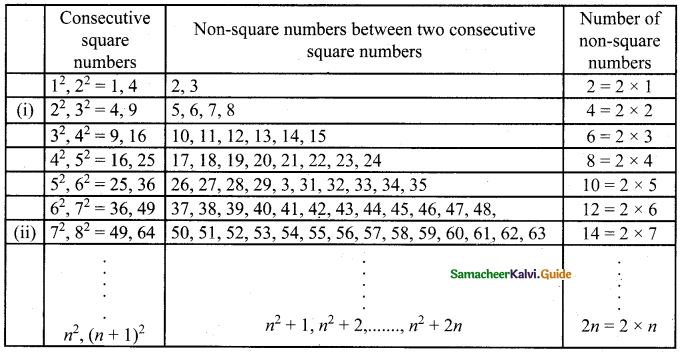Therefore we conclude that there are 2n non-square numbers between two consecutive square numbers.

Think (Text Book Page No. 30)

In this case, if we want to find the smallest factor with which we can multiply or divide 108 to get a square number, what should we do?
108 = 2 × 2 × 3 × 3 = 22 × 32 × 3
If we multiply the factors by 2, then we get
22 × 32 × 3 × 3 = 22 × 32 × 32 = (2 × 3 × 3)2
Which is perfect square.
∴ Again if we divide by 3 then we get 22 × 32 ⇒ (2 × 3)2, a perfect square.
∴ We have to multiply or divide 108 by 3 to get a perfect square.

Try These (Text Book Page No. 32)

Find the square root by long division method.
Question 1.
400√400 = 20

Question 2.
1764√1764 = 42

Question 3.
9801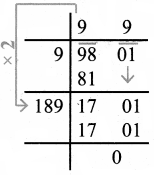√9801 = 66

Try These (Text Book Page No. 32)

without calculating the square root, guess the number of digits in the square root of the following numbers:
Question 1.
14400
14400−−−−−√ = 144×100−−−−−−−−√
= 144−−−√×100−−−√
= 12 × 10
= 120

Question 2.
390625
390625−−−−−−√ = 25×25×25×25−−−−−−−−−−−−−−√
= 25×25−−−−−−√×25×25−−−−−−√
= 25 × 25
= 625

Question 3.
100000000
100000000−−−−−−−−√ = 10000×10000−−−−−−−−−−−√
= 10000−−−−−√×10000−−−−−√
= 100 × 100
= 10,000

Try These (Text Book Page No. 33)

Find the square root of
Question 1.
5.4756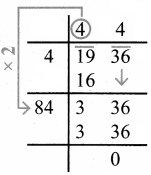Question 2.
19.36Question 3.
116.64Think (Text Book Page No. 33)

Try to fill in the blanks using √ab = √a × √b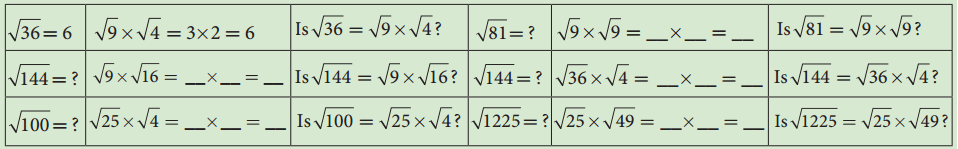Try These (Text Book Page No. 34)

Using this method, find the square root of the numbers 1.2321 and 11.9025.
(i) 1.2321
√1.2321 = 1232110000−−−−√
= 111100 = 1.11

(ii) 11.9025
√11.9025 = 119025√10000√
= 345100 = 3.45

Try These (Text Book Page No. 34)

Write the numbers in ascending order.
Question 1.
4, √14, 5
Squaring all the numbers we get 42, (√14)2, 52 ⇒ 16, 14, 25
∴ Ascending order: 14, 16, 25
Ascending order: √14, 4, 5

Question 2.
7, √65, 8
Squaring 7, √65 and 8 we get 72, (√65)2, 82 ⇒ 49, 65, 64
Ascending order : 49, 64, 65
Ascending order: 7, 8, √65

Try These (Text Book Page No. 37)

Find the ones digit in the cubes of each of the following numbers.
(i) 12
(ii) 27
(iii) 38
(iv) 53
(v) 71
(vi) 84
(i) 12
12 ends with 2, so its cube ends with 8 i.e. ones digit in 123 is 8.

(ii) 27
27 ends with 7, so its cube end with 3. i.e., ones digit in 273 is 3.

(iii) 38
38 ends with 8, so its cube ends with 2 i.e. ones digit in 383 is 2.

(iv) 53
53 ends with 3, so its cube ends with 7. i.e, ones digit in 533 is 7.

(v) 71
71 ends with 1, so its cube ends with 1. i.e. ones digit in 713 is 1

(vi) 84
84 ends with 4, so its cube ends with 4. i.e, ones digit in 843 is 4.

Try These (Text Book Page No. 41)

Expand the following numbers using exponents:
(i) 8120
(ii) 20,305
(iii) 3652.01
(iv) 9426.521
(i) 8120
8120 = (8 × 1000) + (1 × 100) + (2 x×10) + 0 × 1
= (8 × 103) + (1 × 102) + (2 × 101)

(ii) 20,305
20305 = (2 × 10000) + (0 × 1000) + (3 × 100) + (0 × 10) + (5 × 1)
= (2 × 104) + (3 × 102) + 5

(iii) 3652.01
3652.01 = 3000 + 600 + 50 + 2 + 010+1100
= (3 × 1000) + (6 × 100) + (5 × 10) + (2 × 1) + (1 × 1100)
= (3 × 103) + (6 × 102) + (5 × 101) + 2 + (1 × 10-2)

(iv) 9426.521
= (9 × 1000) + (4 × 100) + (2 × 10) + (6 × 1) + (510)+(2100)+(11000)
= (9 × 103) + (4 × 102) + (2 × 101) + 6 + (5 × 10-1) + (2 × 10-2) + (1 × 10-3)

Try These (Text Book Page No. 42)

Verify the following rules (as we did above). Here, a,b are non-zero integers and are any integers.
1. Product of same powers to power of product rule: am × bm = (ab)m
2. Quotient of same powers to power of quotient rule: ambm=(ab)m
3. Zero exponent rule: a0 = 1.
let a = 2; b = 3; m = 2
1. am × bm = 22 × 32
= 4 × 9 = 36 = (2 × 3)2

2. ambm=2232=49=(23)2

3. a0 = 20 = 1.

Try These (Text Book Page No. 44)

Question 1.
Write in standard form: Mass of planet Uranus is 8.68 × 1025 kg.
Mass of Planet Uranus = 86800000000000000000000000 kg
[23 zeros after 88]

Question 2.
Write in scientific notation:
(i) 0.000012005
0.000012005 = 1.2005 × 10-5

(ii) 43 12.345
43 12.345 = 4.312345 × 103

(iii) 0.10524
0.10524 = 1.0524 × 10-1

(iv) The distance between the Sun and the planet Saturn 1.4335 × 1012 miles.

## How to Prepare using Samacheer Kalvi 8th Maths Chapter 1 Numbers Notes PDF?

Students must prepare for the upcoming exams from Samacheer Kalvi 8th Maths Chapter 1 Numbers Notes PDF by following certain essential steps which are provided below.

• Use Samacheer Kalvi 8th Maths Chapter 1 Numbers notes by paying attention to facts and ideas.
• Pay attention to the important topics
• Refer TN Board books as well as the books recommended.
• Correctly follow the notes to reduce the number of questions being answered in the exam incorrectly
• Highlight and explain the concepts in details.

## Frequently Asked Questions on Samacheer Kalvi 8th Maths Chapter 1 Numbers Notes

#### How to use Samacheer Kalvi 8th Maths Chapter 1 Numbers Notes for preparation??

Read TN Board thoroughly, make separate notes for points you forget, formulae, reactions, diagrams. Highlight important points in the book itself and make use of the space provided in the margin to jot down other important points on the same topic from different sources.

#### How to make notes for Samacheer Kalvi 8th Maths Chapter 1 Numbers exam?

Read from hand-made notes prepared after understanding concepts, refrain from replicating from the textbook. Use highlighters for important points. Revise from these notes regularly and formulate your own tricks, shortcuts and mnemonics, mappings etc.
Share: